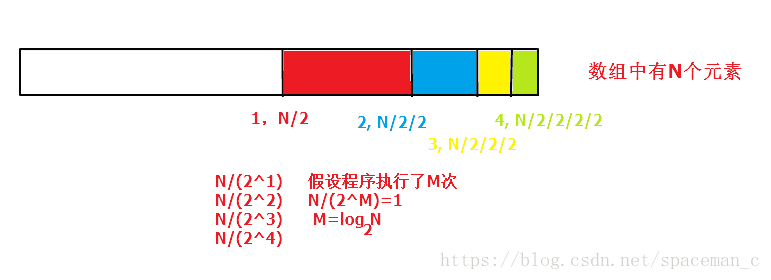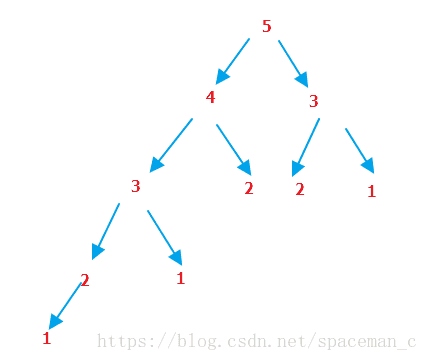# 数据结构时间复杂度进阶篇

2018/10/15 10:02

. 时间复杂度

作用：时间复杂度是度量算法执行时间的长短；（时间复杂度简单的理解就是执行语句的条数。如果有循环和递归，则忽略简单语句，直接算循环和递归的语句执行次数）

时间复杂度用大O渐进表示法表示

时间复杂度的计算：

1，找出执行语句的条数。 如果有循环和递归，则忽略简单语句，直接算循环和递归的语句执行次数；如果算法中有包含嵌套的循环，则执行次数通常是将两个循环次数相乘 ，如果算法中包含并列的循环，则将并且的相加；

2， 将语句执行次数的数量级放入大Ο记号中；

用常数1取代运行时间中的加法常数；
在修改后的运行次数函数中，只保留最高阶项；
如果最高项系数存在且不是1，则去除与这个项相乘的常数；

. 空间复杂度

作用：空间复杂度是度量算法所需存储空间的大小（算法的空间复杂度并不是计算实际占用的空间，而是计算整个算法的辅助空间单元的个数）记做S(n)=O(f(n))。
简单理解就是算法执行时创建的变量（包括临时变量）个数

①忽略常数，用O(1)表示
②递归算法的空间复杂度=递归深度N*每次递归所要的辅助空间
③对于单线程来说，递归有运行时堆栈，求的是递归最深的那一次压栈所耗费的空间的个数，因为递归最深的那一次所耗费的空间足以容纳它所有递归过程。递归是要返回上一层的，所以它所需要的空间不是一直累加起来的

int main()
{
int i = 0;
int j = 0;
int count = 0;

for (i = 0; i < n; i++)
{
for (j = 0; j < n; j++)
{

count++;
}
}//执行的次数是n*n

for (i = 0; i < n; i++)
{
count++;
}//执行的次数是n

int sum = 10;

while (sum--)
{
count++;
}//执行的次数是10
return 0;
}

int Binary_Search(int *dest,int len, int x)
{
int left = 0;
int right =len-1;

while (left <= right)
{
int mid = left + (right - left) / 2;
if (x == *(dest + mid))
{
return mid;
}
else if (x < *(dest + mid))
{
right = mid - 1;

}
else if (x > *(dest + mid))
{
left = mid + 1;
}

}//找到返回下标，没找到返回-1

return -1;
}int fib_num(int x)
{
if (x < 3)
return 1;
else
return fib_num(x - 1) + fib_num(x - 2);
}fib(5)的最深递归层数为5，fib(N)的最深递归层数为N，

int fib_iteration(int n)
{
int a = 1;
int b = 1;
int c = 1;
if (n<2)
{
return n;
}
while (n>2)
{
c = a + b;
a = b;
b = c;
n--;
}
return c;
}

---------------------

0
0 收藏

### 作者的其它热门文章0 评论
0 收藏
0